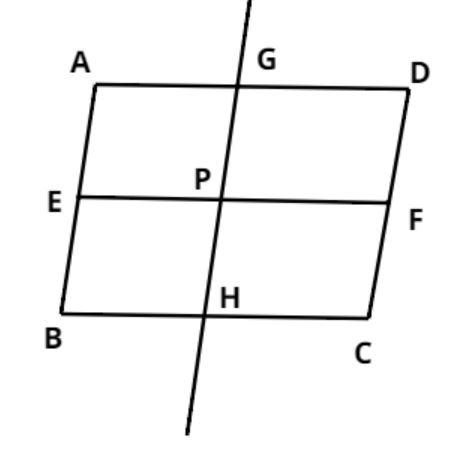Courses
Courses for Kids
Free study material
Free LIVE classes
MoreLIVE
Join Vedantu’s FREE Mastercalss

# ABCD is a parallelogram, E and F are the mid-points of AB and CD respectively. GH is any line intersecting AD, EF and BC at G, P and H respectively. Prove that GP=PH.Verified
262.2k+ views
Hint: In this problem ABCD is a quadrilateral given and we have to prove that for a line GH intersecting AD, EF and BC at some specific points, then GP=PH. If we can prove that P is the midpoint of GH then the required condition will hold true. So use the property of parallelogram that opposite sides are equal and parallel to reach to the solution.Consider ABCD parallelogram as shown above, E and F are mid-points of AB and DC (see figure) and a line GH intersects AD, EF and BC at G, P and H respectively (see figure).
Now since E and F are the midpoints of AB and DC.
$\Rightarrow AE = EB = \dfrac{{AB}}{2}{\text{ & }}DF = FC = \dfrac{{DC}}{2}$………….. (1)
Now as we know opposite sides of a parallelogram are equal.
$\Rightarrow AB = DC{\text{ & }}AD = BC$…………………… (2)
Therefore from equation (1) and equation (2)
$AE = EB = DF = FC = \dfrac{{AB}}{2}$
As we have proved that AE=DF and EB=FC, hence using the property that opposite sides are equal to parallelograms.
We can say that AEFD and EBCF both are parallelograms.
Now the opposite sides of a parallelogram are parallel thus using this property we can say that
$\Rightarrow AD||EF||BC$………………….. (3) As AEFD and EBCF both are parallelograms.
Since E and F are the midpoints of AB and DC, hence P should also be the mid-point of GH by symmetry.
As P is the midpoint of GH hence
$\Rightarrow GP = PH = \dfrac{{GH}}{2}$
Hence proved.

Note: Whenever we face such types of problems the key concept is to have the understanding of basic parallelogram properties, diagrammatic representation of the information being provided in the question always helps understanding the geometry and symmetry in the figures. This concept will help you get the right answer.
Last updated date: 19th Sep 2023
Total views: 262.2k
Views today: 2.62k